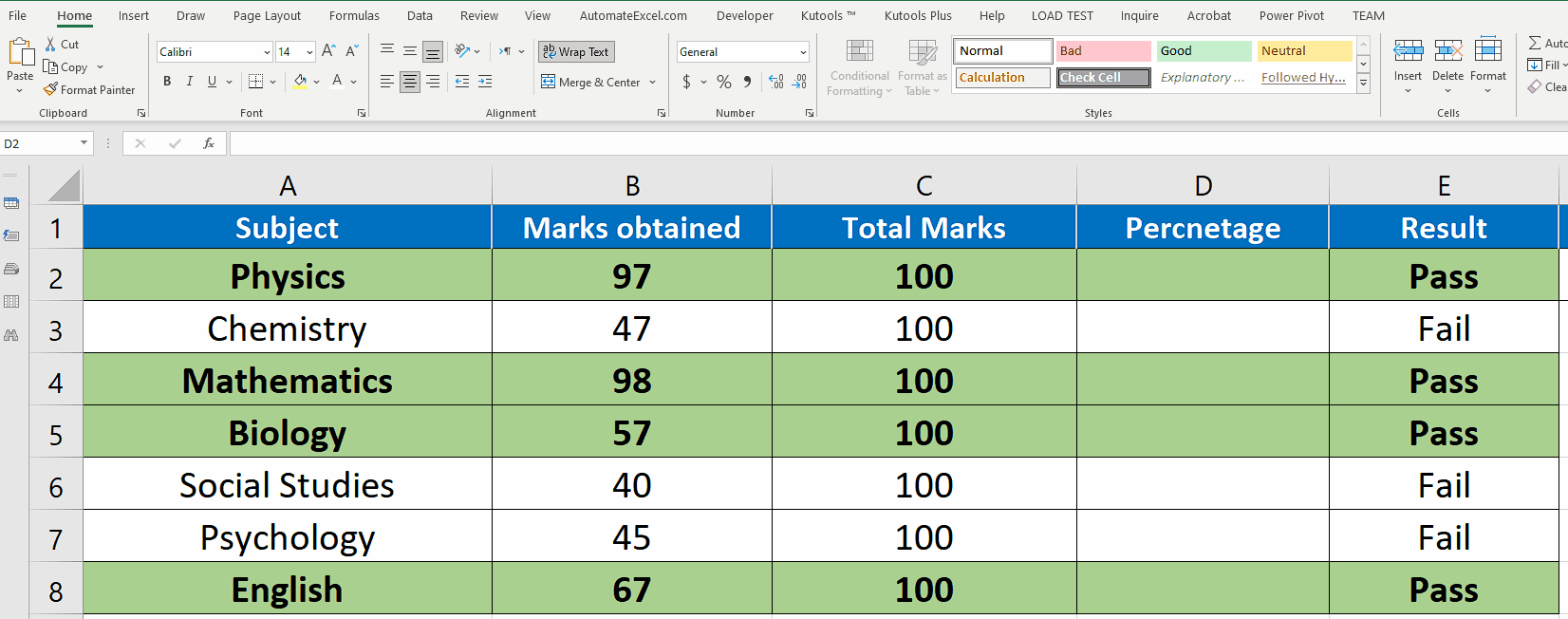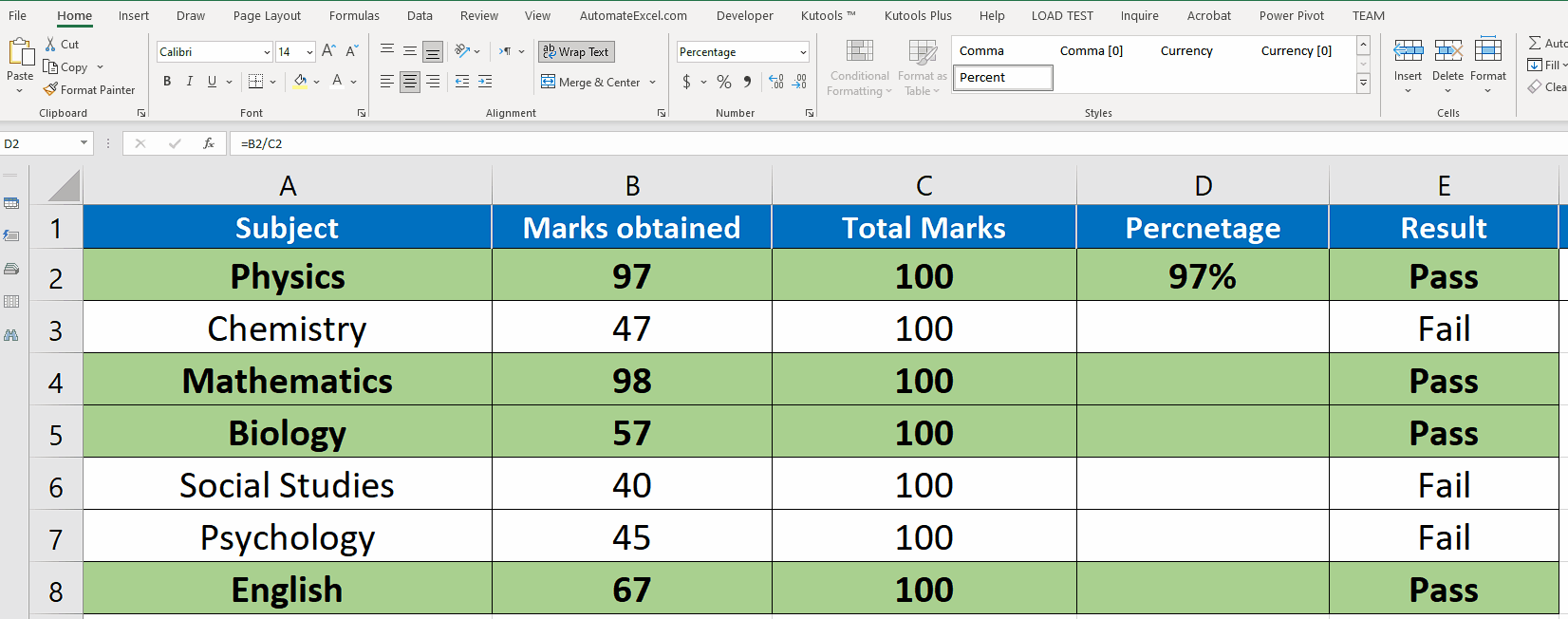# How to copy formula with changing cell reference in Excel

In this tutorial we’ll learn how to write the formula in one cell only and then re-use the same formula in all other cells of the same column or same row by changing the cell references according to the changing rows or columns.

Let’s consider this simple data set which has the details of the marks of a student in various subjects. We’ll calculate the percentage of the student’s marks by using the formula in the first cell and then copy the formula to other cells. Let’s do this step by step.Microsoft Excel is undoubtedly the most powerful tool when it comes to performing calculations, analyzing and manipulating datasets. While performing any calculation on a large data set it is a very common requirement to implement the same formula to all the cells in the same column or row.

### Step 1 – Write the formula in first cell & format cells– To calculate the percentage we’ll use the following simple formula in the cell D2.

=(B2/C2)

– To display the results in percentage format, select all cells in column D and press the percentage sign % on the Home tab in the Number group.

### Step 2 – Copy the formula from first cell with changing references– Now select the fill handle of the first cell, which is the bottom right corner of the cell, and drag down to the last row.

– It will automatically copy the formula to each cell of column D while changing the cell references appropriately to calculate the percentage of each subject correctly.

So this is how you can copy the formula down to all cells with changing references. The benefit of this is the application of the same formula to all desired cells while using the appropriate data to calculate the results properly.